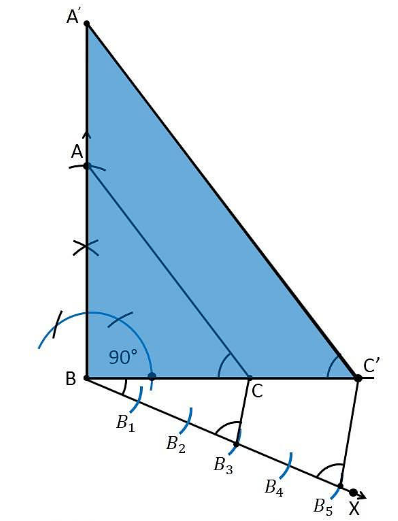# Draw a right triangle in which the sides (other than hypotenuse) are of lengths 4 cm and 3 cm. Then construct another triangle whose sides are 5/3 times the corresponding sides of the given triangle. Give the justification of the construction

To construct a triangle from given conditions

1Let us draw a line segment BC =3 cm.

2. Now measure and draw ∠= 90°

3. Considering B as a centre and draw an arc with a radius of 4 cm and intersects the ray at point B.

4. Now, join the lines AC and the triangle ABC is the required triangle.

5. Draw a ray BX makes an acute angle with BC on the opposite side of vertex A.

6. Locate 5 such as B1, B2 , B3, B4, on the ray BX such that such that BB1 = B1B2 = B2B3= B3B4 = B4B5

7. Join the points B3C.

8. Draw a line through B5 parallel to B3C which intersects the extended line BC at C’.

9. Through C’, draw a line parallel to the line AC that intersects the extended line AB at A’.

10. Therefore, ΔA’BC’ is the required triangle.Justification

since the scale factor is 5/3, we have to justify

A’B = (5/3)AB

BC’ = (5/3)BC

A’C’= (5/3)AC

From the construction, we get A’C’ || AC

In ΔA’BC’ and ΔABC,

∴ ∠ A’C’B = ∠ACB (Corresponding angles)

∠B = ∠B (common)

∴ ΔA’BC’ ∼ ΔABC (From AA similarity criterion)

Since the corresponding sides of the similar triangle are in the same ratio, it becomes

Therefore, A’B/AB = BC’/BC= A’C’/AC

So, it becomes A’B/AB = BC’/BC= A’C’/AC = 5/3